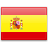S T L Q

S L T Q

Q S T L

S L Q T

S L Q T

S L Q T

S L Q T

Q T L S

S L T Q

L T Q S

S T L Q

S L Q T

S T L

S L T

T Q L S

L T S

L T S

T L S

T L S